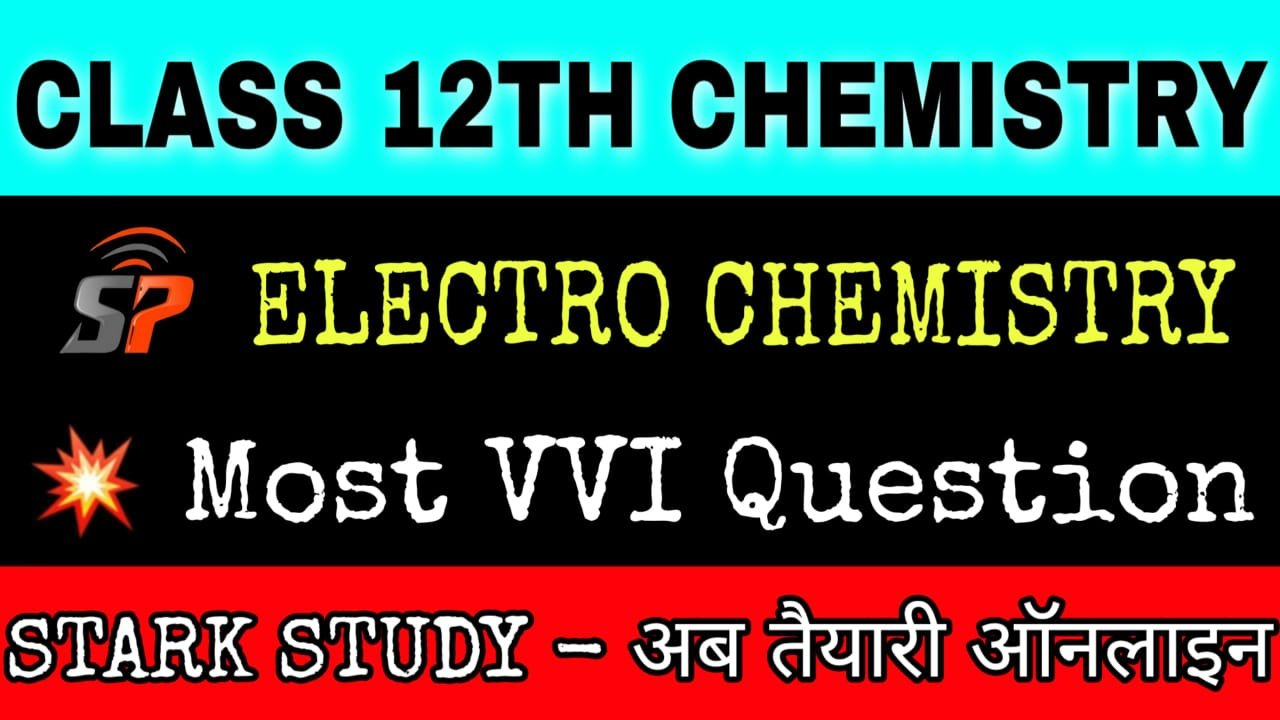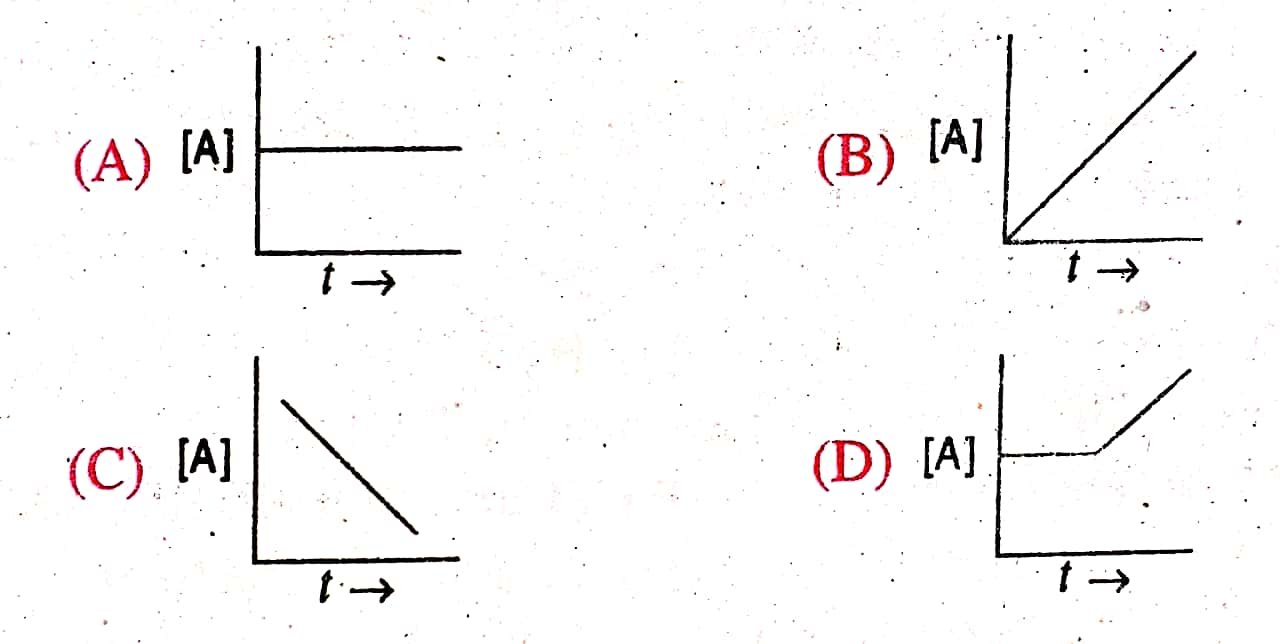12th Board Chemistry Most VVI Objective Question Chemical Kinetics Chapter

4. CHEMICAL KINETICS

1. In any reaction, catalyst:

(A) retards the rate of reaction

(B) increases the rate of reaction

(C) both increases and decreases the rate of reaction

(D) none of these

2. In chemical equation H2(g) + I2 (g) ⇔ 2HI (g) the equilibrium constant Kp depends on:

(A) total pressure

(B) catalyst used

(C) amount of H2 and I2

(D) temperature

3. If the rate of a reaction is expressed by, Rate = k[A]2 [B], the order of reaction will be:

(A) 2

(B) 3

(C) 1

(D) 0

4. The rate constant of a reaction depends upon

(A) temperature of the reaction

(B) extent of the reaction

(C) initial concentration of the reactants

(D) the time of completion of reaction

5. The unit of rate and rate constant are same for a :

(A) zero order reaction

(B) first order reaction

(C) second order reaction

(D) third order reaction

6. For a unimolecular reaction :

(A) the order and molecularity of the slowest step are equal to one

(B) molecularity of the reaction can be zero, one or two

(C) more than one reacting species are involved in one step

(D) molecularity of the reaction can be determined only experimentally

7. The overall rate of a reaction is governed by

(A) the rate of fastest intermediate step

(B) the sum total of the rates of all intermediate steps.

(C) the average of the rates of all the intermediate steps

(D) the rate of slowest intermediate step

8. H2 (g) + Cl2 (g) → 2 HCI (g). The order of this reaction is :

(A) Zero order

(B) First order

(C) Second order

(D) None of these

9. For a reaction if activation energies for forward and backward reactions are the same, then :

(A) ΔH = 0

(B) ΔS = 0

(C) The order is zero

(D) None of these

10. Radioactive disintegration is an example of :

(A) zero order reaction

(B) first order reaction

(C) second order reaction

(D) third order reaction

11. t1/2 for first order reaction is :

(A) 0.6 / k

(B) 0.693 / k

(C) 0.683 / k

(D) 0.10 / k

12. The rate at which a substance reacts, depends upon its

(A) Atomic Mass

(B) Equivalent Mass

(C) Molecular Mass

(D) Active Mass

13. Half life period of first order reaction is independent of :

(A) Initial concentration of reactant

(B) Temperature

(C) Pressure

(D) None of these

14. Which represents a zero order reaction ?